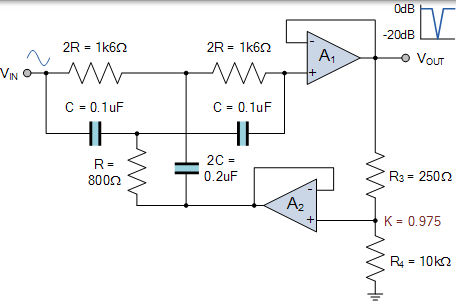# 带阻滤波器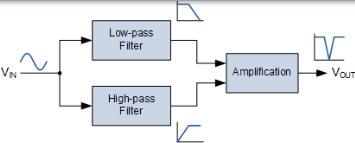### 带阻滤波器响应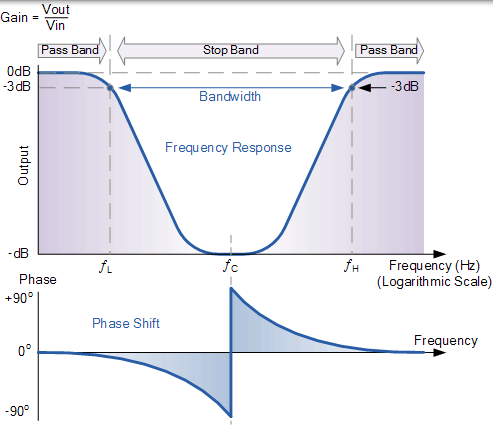### 典型的带阻滤波器配置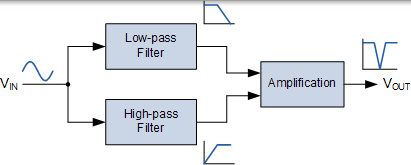### 带阻滤波器特性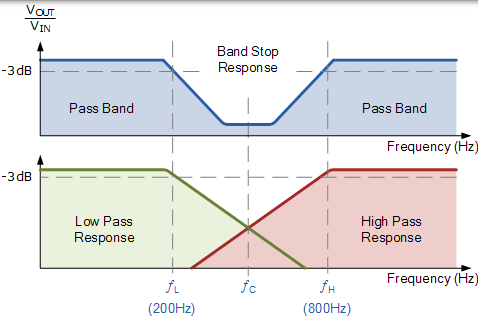### 带阻滤波电路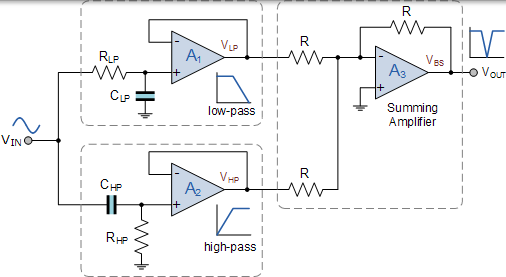## 带阻滤波器示例 No1

$$f = \frac { 1 } { 2 \pi R C } \textrm {Hz}$$

### 低通滤波器部分

$$\begin{array} { l } { f _ { \mathrm { L } } = \frac { 1 } { 2 \pi \mathrm { R } _ { \mathrm { L } } \mathrm { C } } = 200 \mathrm { Hz } \text { and } \mathrm { C } = 0.1 \mathrm { UF } } \\ { \therefore \mathrm { R } _ { \mathrm { L } } = \frac { 1 } { 2 \pi \times 200 \times 0.1 \times 10 ^ { - 6 } } = 7958 \Omega \text { or } 8 \mathrm { k } \Omega } \end{array}$$

### 高通滤波器部分

$$\begin{array} { l } { f _ { \mathrm { H } } = \frac { 1 } { 2 \pi \mathrm { R } _ { \mathrm { H } } \mathrm { C } } = 800 \mathrm { Hz } \text { and } \mathrm { C } = 0.1 \mathrm { uF } } \\ { \therefore \mathrm { R } _ { \mathrm { H } } = \frac { 1 } { 2 \pi \times 800 \times 0.1 \times 10 ^ { - 6 } } = 1990 \Omega \text { or } 2 \mathrm { k } \Omega } \end{array}$$

$$\begin{array} { l } { f _ { \mathrm { C } } = \sqrt { f _ { \mathrm { L } } \times f _ { \mathrm { H } } } = \sqrt { 200 \times 800 } = 400 \mathrm { Hz } } \\ { f _ { \mathrm { B } W } = f _ { \mathrm { H } } - f _ { \mathrm { L } } = 800 - 200 = 600 \mathrm { Hz } } \\ { \mathrm { Q } = \frac { f _ { \mathrm { C } } } { f _ { \mathrm { BW } } } = \frac { 400 } { 600 } = 0.67 \mathrm { Or } - 3.5 \mathrm { dB } } \end{array}$$

### 带阻滤波器设计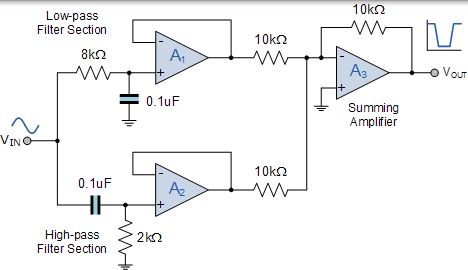## 陷波滤波器

### 基本的 Twin-T 陷波滤波器设计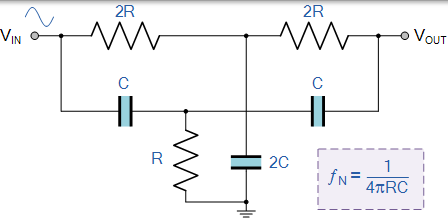### Twin-T 陷波滤波器方程

$$f _ { N } = \frac { 1 } { 4 \pi R C }$$

### 单运放双 T 陷波滤波器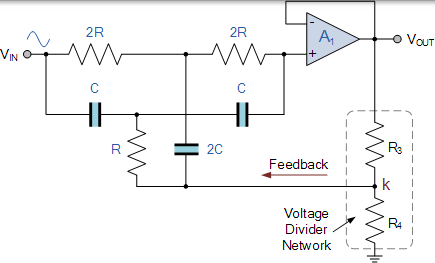$$\mathrm { K } = \frac { \mathrm { R } _ { 4 } } { \mathrm { R } _ { 3 } + \mathrm { R } _ { 4 } } = 1 - \frac { 1 } { 4 \mathrm { Q } }$$

Q 的值由 R3 和 R4 电阻比决定，但如果我们想让 Q 完全可调，我们可以用一个电位器代替这两个反馈电阻，并将其馈入另一个运放缓冲器以增加负增益。另外，为了获得给定频率下的最大陷波深度，可以去掉电阻 R3 和 R4，并且 R 和 2C 的连接点直接连接到输出端。

## 带阻滤波器示例 No2

1. 计算给定电容 0.1uF 的 R 值

$$\begin{array} { l } { \mathrm { R } = \frac { 1 } { 4 \pi f _ { \mathrm { N } } \mathrm { C } } = \frac { 1 } { 4 \pi \times 1000 \times 0.1 \times 10 ^ { - 6 } } } \\ { \therefore \mathrm { R } = 795 \Omega \text { or } 800 \Omega } \end{array}$$

1. 计算 Q 值

$$\mathrm { Q } = \frac { f _ { \mathrm { N } } } { \mathrm { B } W } = \frac { 1000 } { 100 } = 10$$

1. 计算反馈系数 k

$$\mathrm { k } = 1 - \frac { 1 } { 4 \mathrm { Q } } = 1 - \frac { 1 } { 4 \times 10 } = 0.975$$

1. 计算电阻器 R3 和 R4 的值

$$\mathrm { k } = 0.975 = \frac { \mathrm { R } _ { 4 } } { \mathrm { R } _ { 3 } + \mathrm { R } _ { 4 } } \\ \textrm{假设 } \mathrm {R_{4} = 10 k \Omega }， \\ { \mathrm { R } _ { 3 } = \mathrm { R } _ { 4 } - 0.975 \mathrm { R } _ { 4 } = 10000 - 0.975 \times 10000 } \\ { \therefore \mathrm { R } _ { 3 } = 250 \Omega }$$

1. 以分贝为单位计算预期的陷波深度，dB

$$\begin{array} { l } { \frac { 1 } { Q } = \frac { 1 } { 10 } = 0.1 } \ { f _ { N d B } = 20 \log ( 0.1 ) = - 20 \mathrm { d } B } \end{array}$$

### 陷波滤波器设计